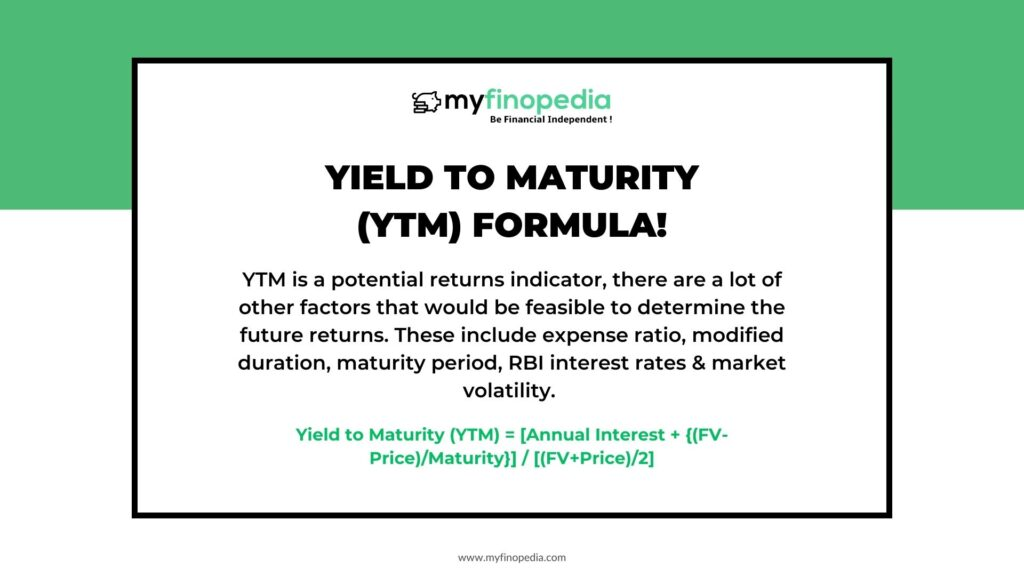# How to Find Yield to Maturity (YTM)?For Long-term investors a Yield to Maturity (YTM) works as a crucial factor. It indicates the maximum potential returns of a debt fund that one acquires until it gets matured. As the predictability is low in this case, the returns can be calculated by using yield to maturity. The simple formula helps a lot in determining what is the actual cost you get by investing in a bond through years.

Let’s look upon the major factors that depend on the fund. They are as follows:-

Any debt portfolio can include a single or multiple bonds, As a result, the average of the entire bond investment is calculated to determine the threshold for a significant number of years. Here are the key factors that are required:-

FV = Face value denotes the initial or the price at issue of the bond in respect of a significant amount of years.

Annual Rate of Interest is the payout that is decided or issued by the bond owner.

Maturity of Bond is the number of years the bond is held to get matured.

Market Value determines the ongoing market price or volatility of the bond, which is being traded.

Now the formula becomes-

Yield to Maturity (YTM) = [Annual Interest + {(FV-Price)/Maturity}] / [(FV+Price)/2]

For example, if one bond has FV= 500/- ; Annual coupon rate = 5%; Annual Payout of Interest= 50/- ; Tenure of Maturity= 6 years & market value = 450/-

Then, according to the formula,

Yield to Maturity (YTM) = [50 + (500-450)/6} / (500+450)/2] = 12.46

In case of multiple bonds, one has to measure the weightage calculating the average of all the single bonds.

While YTM is a potential returns indicator, there are a lot of other factors that would be feasible to determine the future returns. These include expense ratio, modified duration, maturity period, RBI interest rates & market volatility. Thus, one has to critically understand to estimate broader apprehension of the returns.